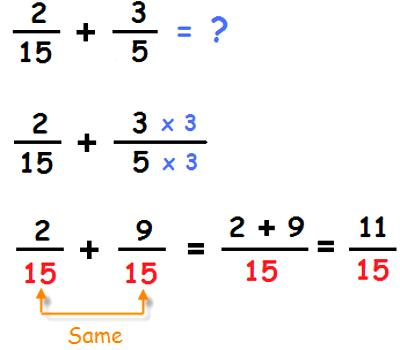Comparing Fractions with Different Denominators

A Fraction consists of two numbers separated by a line.

The top number (or numerator) tells how many fractional pieces there are. The fraction 3/8 indicates that there are three pieces.

The denominator of a fraction tells how many pieces an object was divided into. The fraction 3/8 indicates that the whole object was divided into 8 pieces.

If the numerators of two fractions are the same, the fraction with the smaller denominator is the larger fraction.

For example 5/8 is larger than 5/16 because each fraction says there are five pieces but if an object is divided into 8 pieces, each piece will be larger than if the object were divided into 16 pieces. Therefore, five larger pieces are more than five smaller pieces.Explanation

Subtracting fractions with like denominators is easy, but when the fractions have unlike denominators, it can take a few steps to make them have the same denominator so that you can subtract them. These few steps can take a little while, but once you get the hang of it, you’ll be able to subtract fractions in no time at all. If you want to know how to do it, just follow these steps.

Find the denominators of the fractions. If you want to subtract fractions, then the first thing you have to do is to make sure that they have like denominators. The numerator is the number on the top of the fraction and the denominator is the number on the bottom. In the example, 3/4 – 1/3, the two denominators of the fractions are 4 and 3. Circle them.

Find the least common multiple (LCM) of the denominators. The LCM of two numbers is the smallest number that is evenly divisible by both numbers. You’ll need to find the LCM of 4 and 3, which will give you the lowest common denominator (LCD) of the fraction.

Make the numerators of the fractions match their new denominators. Now that you know that the LCM of 4 and 3 is 12, you can think of 12 as the new denominator of the fractions. But to make the fractions equivalent, you’ll have to multiply their numerators by a number that would give them the same value with their new denominators.

Write the new numerators over the lowest common denominator. Now that you know that the lowest common multiple of 4 and 3 is 12, you can say that the lowest common denominator of the fractions 1/3 and 3/4 is 12.

Subtract the numerators. Once you have written the new numerators over the LCD, you are ready to subtract. Simply subtract the numerators in the appropriate order; do not do anything to the denominator.

Simplify your answer. Once you have your answer, check to see if you can simplify it. If the numerator and denominator can be divided by the same number, divide them by that number. Remember that fractions are proportions, so whatever you do to the numerator, you must also do to the denominator.

Information Source: